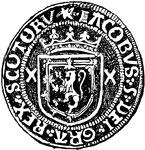### Écu, Obverse

The obverse side of an écu of James V of Scotland, a Scotch gold coin also called a crown.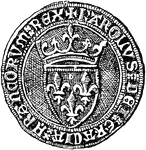### Écu, Obverse

The obverse side of the écu d'or of Charles VI, king of France.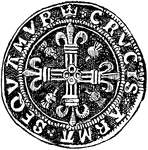### Écu, Reverse

The reverse side of an écu of James V of Scotland, a Scotch gold coin also called a crown.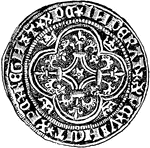### Écu, Reverse

The reverse side of the écu d'or of Charles VI, king of France.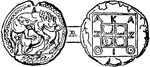### Coin of Acanthus

The coin of the ancient city Acanthus in Egypt.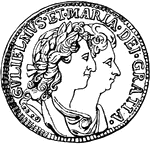### Accolated

"Accolated shilling of William III and Mary(size of the original)"-Whitney, 1902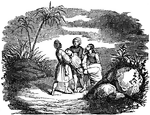### The Body of Ananias is Taken to be Buried

"And Ananias hearing these words fell down and gave up the ghost: and great fear came upon all that…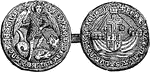### Angel

The Angel of Edward IV, a gold coin named after the picture stamped on it of the angel Michael.### Coin of Anne

"Coin of Anne." — Lardner, 1885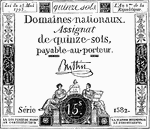### Assignat

"After appropriating to national purposes the land belonging to the church, the French National Assembly,…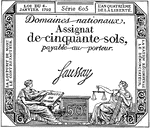### An Assignat

A type of monetary instrument used during the French Revolution.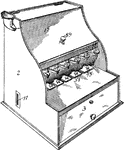### Coin Assorter

This tool is used for sorting coins, A coin is a piece of hard material that is standardized in weight,…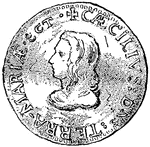### Baltimore Shilling

Colonial Currency, Lord Baltimore shilling.### Bank Draft

Common form of bank draft, or check for five hundred dollars.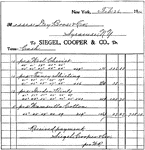### Receipted Bill for Dry Goods

A company's bill for dry goods with a breakdown of costs and a note of receipt at the bottom.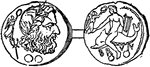### Brindaban

Coin of Brindaban, a town in Northwest India.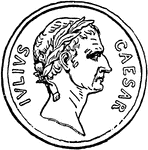### Caesar Coin

Julius Caesar issued Roman coins bearing his own image.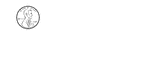### 1 Cent

Groups of change with totals from 1 to 100 cents using the least amount of coins.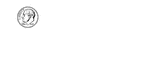### 10 Cents

Groups of change with totals from 1 to 100 cents using the least amount of coins.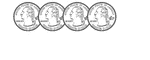### 100 Cents

Groups of change with totals from 1 to 100 cents using the least amount of coins.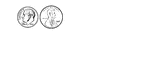### 11 Cents

Groups of change with totals from 1 to 100 cents using the least amount of coins.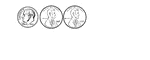### 12 Cents

Groups of change with totals from 1 to 100 cents using the least amount of coins.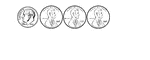### 13 Cents

Groups of change with totals from 1 to 100 cents using the least amount of coins.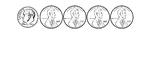### 14 Cents

Groups of change with totals from 1 to 100 cents using the least amount of coins.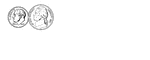### 15 Cents

Groups of change with totals from 1 to 100 cents using the least amount of coins.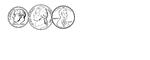### 16 Cents

Groups of change with totals from 1 to 100 cents using the least amount of coins.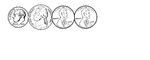### 17 Cents

Groups of change with totals from 1 to 100 cents using the least amount of coins.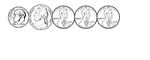### 18 Cents

Groups of change with totals from 1 to 100 cents using the least amount of coins.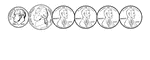### 19 Cents

Groups of change with totals from 1 to 100 cents using the least amount of coins.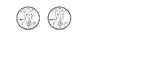### 2 Cents

Groups of change with totals from 1 to 100 cents using the least amount of coins.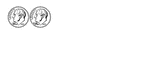### 20 Cents

Groups of change with totals from 1 to 100 cents using the least amount of coins.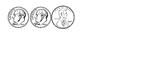### 21 Cents

Groups of change with totals from 1 to 100 cents using the least amount of coins.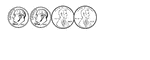### 22 Cents

Groups of change with totals from 1 to 100 cents using the least amount of coins.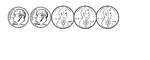### 23 Cents

Groups of change with totals from 1 to 100 cents using the least amount of coins.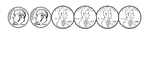### 24 Cents

Groups of change with totals from 1 to 100 cents using the least amount of coins.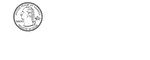### 25 Cents

Groups of change with totals from 1 to 100 cents using the least amount of coins.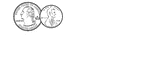### 26 Cents

Groups of change with totals from 1 to 100 cents using the least amount of coins.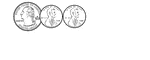### 27 Cents

Groups of change with totals from 1 to 100 cents using the least amount of coins.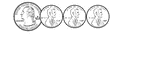### 28 Cents

Groups of change with totals from 1 to 100 cents using the least amount of coins.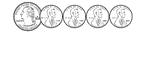### 29 Cents

Groups of change with totals from 1 to 100 cents using the least amount of coins.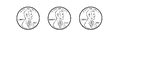### 3 Cents

Groups of change with totals from 1 to 100 cents using the least amount of coins.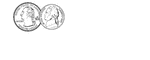### 30 Cents

Groups of change with totals from 1 to 100 cents using the least amount of coins.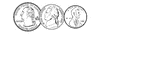### 31 Cents

Groups of change with totals from 1 to 100 cents using the least amount of coins.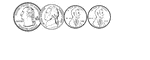### 32 Cents

Groups of change with totals from 1 to 100 cents using the least amount of coins.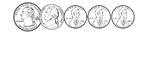### 33 Cents

Groups of change with totals from 1 to 100 cents using the least amount of coins.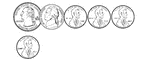### 34 Cents

Groups of change with totals from 1 to 100 cents using the least amount of coins.### 35 Cents

Groups of change with totals from 1 to 100 cents using the least amount of coins.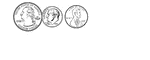### 36 Cents

Groups of change with totals from 1 to 100 cents using the least amount of coins.### 37 Cents

Groups of change with totals from 1 to 100 cents using the least amount of coins.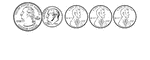### 38 Cents

Groups of change with totals from 1 to 100 cents using the least amount of coins.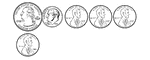### 39 Cents

Groups of change with totals from 1 to 100 cents using the least amount of coins.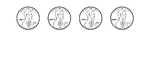### 4 Cents

Groups of change with totals from 1 to 100 cents using the least amount of coins.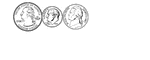### 40 Cents

Groups of change with totals from 1 to 100 cents using the least amount of coins.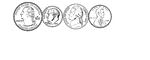### 41 Cents

Groups of change with totals from 1 to 100 cents using the least amount of coins.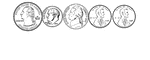### 42 Cents

Groups of change with totals from 1 to 100 cents using the least amount of coins.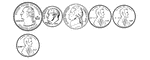### 43 Cents

Groups of change with totals from 1 to 100 cents using the least amount of coins.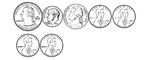### 44 Cents

Groups of change with totals from 1 to 100 cents using the least amount of coins.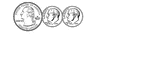### 45 Cents

Groups of change with totals from 1 to 100 cents using the least amount of coins.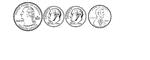### 46 Cents

Groups of change with totals from 1 to 100 cents using the least amount of coins.